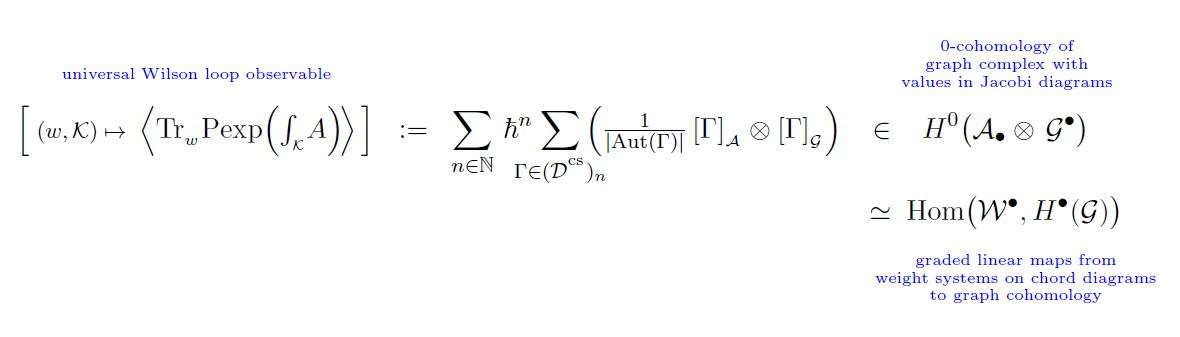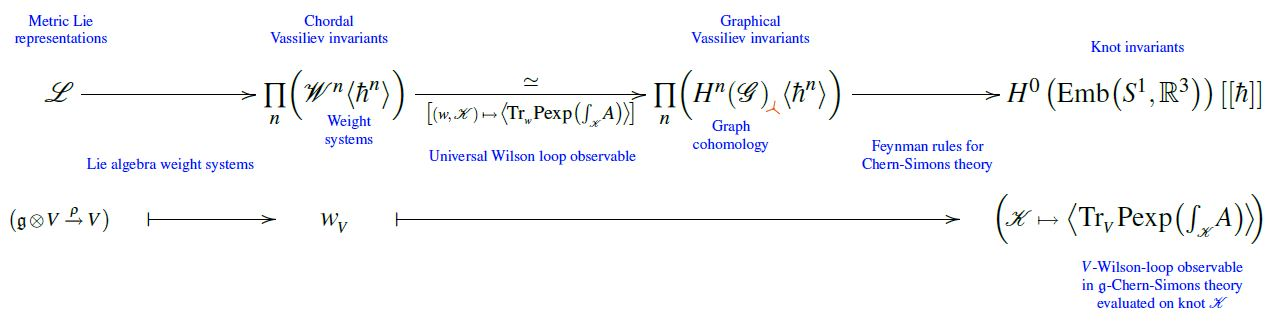# nLab weight systems are cohomology of knot graph complex

Contents

### Context

#### Knot theory

knot theory

Examples/classes:

Types

knot invariants

Related concepts:

category: knot theory

# Contents

## Idea

The cochain cohomology of the framed knot graph complex (sometimes called the “Wilson graph complex”) spanned by trivalent graphs coincides with the space of framed weight systems on Jacobi diagrams, equivalently on round chord diagrams:

$\underset{ \phantom{a} \atop { {\color{blue}framed\;weight\;systems\;on} \atop {\color{blue}round\;chord\;diagrams} } }{ \mathcal{W}^\bullet } \;\simeq\; \underset{ \phantom{a} \atop { {\color{blue}\weight\;systems\;on} \atop {\color{blue}Jacobi\;diagrams} } }{ \mathcal{W}^\bullet } \underoverset { \;\;\;\simeq\;\;\; } { (w,\mathcal{K}) \mapsto \left\langle Tr_{{}_{w}} \text{P}\exp \left( \int_{\mathcal{K}} A \right) \right\rangle } { \longrightarrow } \underset{ \mathclap{ {\phantom{a}} \atop { {\color{blue}cohomology\;of\;knot\;graph\;complex} \atop {\color{blue}spanned\;by\;trivalent\;graphs} } } }{ H^{\bullet} \big( \mathcal{G} \big)_3 }$

This statement is made explicit as CCRL 02, Prop. 7.6, where it is noticed that this is implicit in statement and proof of AF 96, Theorem 1 (where in turn the argument is attributed to Kohno 94!)

Moreover:

If $w \in \mathcal{W}$ is a weight system and $D \in \mathcal{D}$ is a Jacobi diagram such that $w(D) \neq 0$, then its image $\left\langle Tr_{{}_{w}} \text{P}\exp \left( \int_{(-)} A \right) \right\rangle$ under the above isomorphism contains a non-vanishing multiple of $D$ as a summand.

This is made explicit as CCRL 02, Remark 7.7 and again this is implicit in the statement of AF 96, Theorem 1.

What AF 96 explicitly construct is a universal Vassiliev invariant, which they identify with the un-traced Wilson loop observable

$\mathcal{K} \mapsto \left\langle Tr_{{}_{(w)}} \text{P}\exp \left( \int_{\mathcal{K}} A \right) \right\rangle$

## Ingredients

Write

(1)$\mathcal{D}^{cs} \;\in\; Set^{\mathbb{N}}$

for the graded set of isomorphism classes of trivalent framed knot graphs – Feynman diagrams for Chern-Simons theory in the presence of Wilson loops, called “Wilson graphs” AF 96, Section 1, slightly differing from the un-framed knot graphs in CCRL 02.

By definition, a graph $\Gamma \in \mathcal{D}^{cs}$ must have even number of vertices, and its degree is half that number (AF 96, (2.9))

$\Gamma \;\in\; \mathcal{D}^{cs}_{(\# Vert_\Gamma)/2} \,.$

For any $\Gamma \in \mathcal{D}^{cs}$ write

$Aut(\Gamma) \;\in\; Grp$

for its automorphism group, a finite group whose order we denote by

(2)$\left\vert Aut(\Gamma)\right\vert \;\in\; \mathbb{N} \,.$

Write

(3)$\mathcal{G}^\bullet \;\in\; Ch^\bullet(\mathbb{R})$

for the framed knot graph complex and

$H^\bullet(\mathcal{G})_3 \subset H^\bullet(\mathcal{G})$

for the sub-vector space of its cohomology spanned by cocycles made of trivalent graphs.

Write

(4)$\mathcal{A}_\bullet \;\coloneqq\; Span \left( \mathcal{D}^t \right)/(STU) \; \in \; Vect_\bullet(\mathbb{R})$

Also write

(5)$\array{ && \mathcal{D}^{cs} \\ & {}^{\mathllap{ [-]_{{}_{\mathcal{A}}} }} \swarrow && \searrow^{\mathrlap{ [-]_{\mathcal{G}} }} \\ \mathcal{A}_\bullet && && \mathcal{G}^\bullet }$

for the functions that send a graph to the defining basis vector that it represents in these vector spaces, respectively.

The space $\mathcal{A}_\bullet$ is the graded linear dual of the space of weight systems

$\mathcal{W}^\bullet \;\coloneqq\; (\mathcal{A}_\bullet)^\ast \,.$

Hence if we regard

$\mathcal{A}_\bullet \;=\; (\mathcal{A}^{-\bullet}, d= 0)$

as a cochain complex in non-positive degree with vanishing differential, then its tensor product of cochain complexes with the knot graph complex is the cochain complex whose closed elements are the graded linear maps from $\mathcal{W}^\bullet$ to the cochain cohomology $H^\bullet(GraphCplx)$ of the knot graph complex:

(6)$H^0 \big( \mathcal{A}_\bullet \otimes \mathcal{G}^\bullet \big) \;\simeq\; Hom \big( \mathcal{W}^\bullet , H^\bullet(\mathcal{G}) \big)$

## Statement

###### Proposition

The element

(7)$\left\langle Tr_{(-)} \text{P}\exp \left( \int_{(-)} A \right) \right\rangle \;\coloneqq\; \underset{ n \in \mathbb{N} }{\sum} \hbar^n \underset{ \Gamma \in \mathcal{D}^{cs}_n }{\sum} \left( \frac{1}{\left\vert \Gamma\right\vert} \, [\Gamma]_{\mathcal{A}} \otimes [\Gamma]_{\mathcal{G}} \right) \; \in \;\; \mathcal{A}_\bullet \otimes \mathcal{G}^\bullet$

(hence the sum over Feynman diagrams (1) of the tensor product of their images (5) in Jacobi diagrams modulo the STU-relations (4) and in the knot graph complex (3), respectively, weighted by the inverse order of their automorphism group (2) )

is closed

$d_{\mathcal{G}} \left\langle Tr_{(-)} \text{P}\exp \left( \int_{(-)} A \right) \right\rangle \;=\; 0$

This is AF 96, Theorem 1.

Hence (7) defines a cochain cohomology-class

\begin{aligned} \left\langle Tr_{(-)} \text{P}\exp \left( \int_{(-)} A \right) \right\rangle \;\coloneqq\; H^0 \big( \mathcal{A}_\bullet \otimes \mathcal{G}^\bullet \big) \\ & \;\simeq\; Hom \big( \mathcal{W}^\bullet , H^\bullet(\mathcal{G}) \big) \end{aligned}

and hence, by (6), it defines a graded linear function

$\mathcal{W}^\bullet \overset{ \left\langle Tr_{(-)} \text{P}\exp \left( \int_{(-)} A \right) \right\rangle }{ \longrightarrow } H^\bullet(\mathcal{G})_3 \hookrightarrow H^\bullet(\mathcal{G})$

from weight systems on Jacobi diagrams (equivalently on round chord diagrams) to the cochain cohomology of the framed knot graph complex spanned by trivalent graphs.

According to CCRL 02, Prop. 7.6 this map is a bijection.

To see this, use 1) AF 96, Theorem 5, Condition U2 to find that the map is an injection, and 2) the fact that weight systems are associated graded of Vassiliev invariants.

In summary we have the following situation:graphics from Sati-Schreiber 19c

• Toshitake Kohno, Vassiliev invariants and de Rham complex on the space of knots,

In: Yoshiaki Maeda, Hideki Omori, Alan Weinstein (eds.), Symplectic Geometry and Quantization, Contemporary Mathematics 179 (1994): 123-123 (doi:10.1090/conm/179)

• Daniel Altschuler, Laurent Freidel, Vassiliev knot invariants and Chern-Simons perturbation theory to all orders, Commun. Math. Phys. 187 (1997) 261-287 (arXiv:q-alg/9603010)

• Alberto Cattaneo, Paolo Cotta-Ramusino, Riccardo Longoni, Configuration spaces and Vassiliev classes in any dimension, Algebr. Geom. Topol. 2 (2002) 949-1000 (arXiv:math/9910139)

Computation of the perturbative Wilson loop observable (universal Vassiliev invariant) of the unknot (“Wheels theorem”):

following